## # 前言

Hey，各位小伙伴，这次怎么来玩一下, 如何使用 Django 执行原生 SQL。

## # 起因

• extra
• raw
• django connection

## # 表结构

``````class Book(models.Model):
title = models.CharField(verbose_name="书名", max_length=32)
describe = models.TextField(verbose_name="描述")
author = models.CharField(verbose_name="作者", max_length=32)
publisher = models.CharField(verbose_name="出版社", max_length=32)
publisher_date = models.DateField(verbose_name="publisher")
``````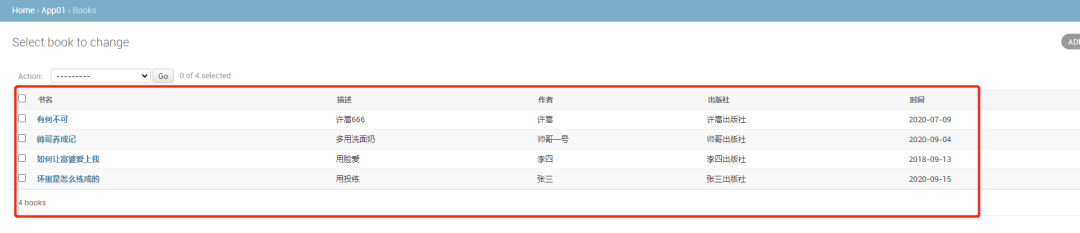extra 方式

## # raw 方式

``````models.表名.objecs.raw(sql)
models.表名.objecs.raw(sql,[参数1,参数2])
``````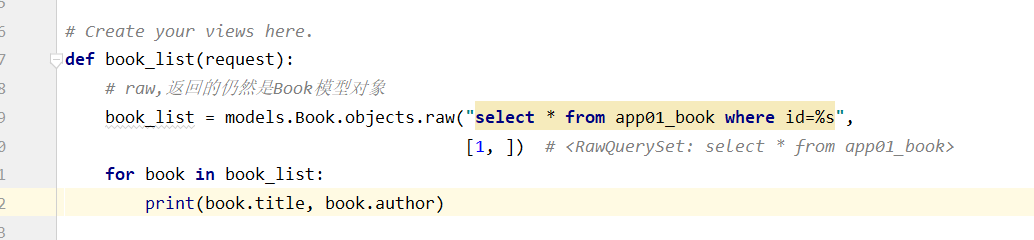## # 真正的原生 sql 方式

``````from django.db import connection
def book_list(request):

cursor = connection.cursor()
print(type(cursor))
cursor.execute("select * from app01_book where id=%s", [1, ])
raw = cursor.fetchall()
print(raw)
``````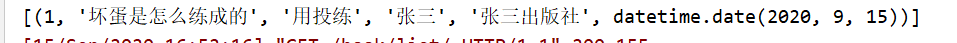## # 执行原生 sql 并且返回成 dict

``````def query_all_dict(sql, params=None):
'''
查询所有结果返回字典类型数据
:param sql:
:param params:
:return:
'''
with connection.cursor() as cursor:
if params:
cursor.execute(sql, params=params)
else:
cursor.execute(sql)
col_names = [desc for desc in cursor.description]
row = cursor.fetchall()
rowList = []
for list in row:
tMap = dict(zip(col_names, list))
rowList.append(tMap)
return rowList
``````

``````def query_one_dict(sql, params=None):
"""
查询一个结果返回字典类型数据
:param sql:
:param params:
:return:
"""
with connection.cursor() as cursor:
if params:
cursor.execute(sql, params=params)
else:
cursor.execute(sql)
col_names = [desc for desc in cursor.description]
row = cursor.fetchone()
tMap = dict(zip(col_names, row))
return tMap
``````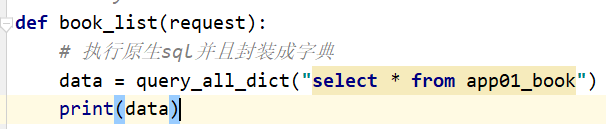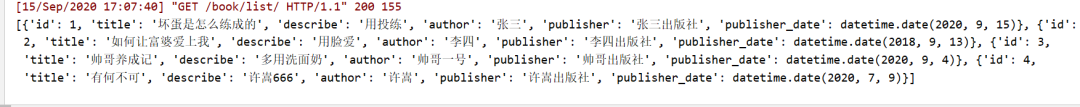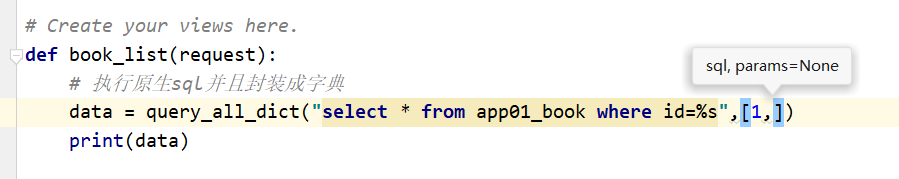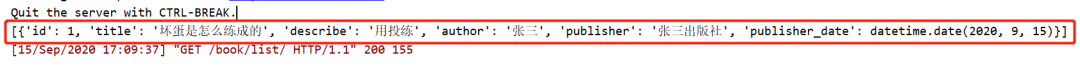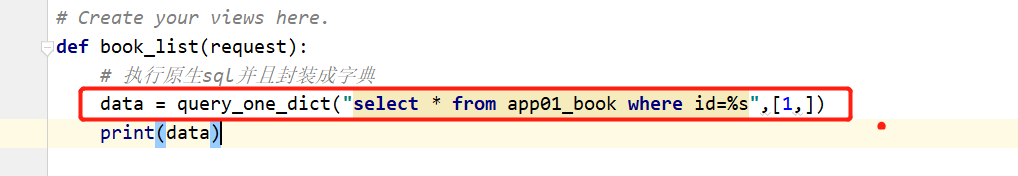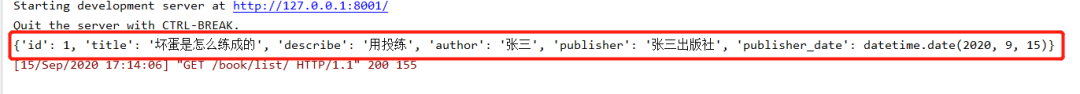## # 上述总结

django 中执行原生 sql 有 3 种方式,`extra`,`raw`,`from django.db import connection`

## # 项目代码

``````django_exec_sql.zip
``````

**-----**---**---****---**--**---********** End **-----**--------**-----**-****/ 今日留言主题 /